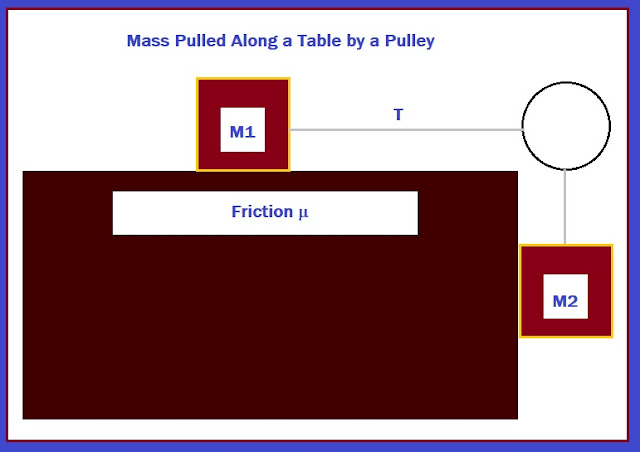## Sunday, July 8, 2018

### Fun with the Radio Shack EC-4004

Fun with the Radio Shack EC-4004

Complex Number Multiplication

(a + bi) * (c + di) = (a*c – b*d) + (b*c + a*d)i

Store values in the following memory registers before running the program:

K1 = a
K2 = b
K3 = c
K4 = d

Output is stored in registers K5 (real part) and K6 (imaginary part).

Program:

Kout 1
*
Kout 3
-
Kout 2
*
Kout 4
=
Kin 5
HLT
Kout 2
*
Kout 3
+
Kout 1
*
Kout 4
=
Kin 6

Example:

(-8 + 3i)*(6 + 3i)   (K1 = -8, K2 = 3, K3 = 6, K4 = 3)

Result:  -57 – 6i (K5 = -57, K6 = -6)]

Product of Integers

The following program will between and b:  Product = a * (a + 1) * (a + 2) * … * b

P = Π n from n = a to b

Store values in the following memory registers before running the program:

K1 = a
M = b
K2 = 1 (store 1 in K2 each time)

Program:

Kout 1
Kin* 2
1
Kin+ 1
Kout 1
X ≤ M
Kout 2

Example:  Π n from n = 5 to 8:  P = 5 * 6 * 7 * 8

Store 5 in K1, 8 in M (Inv Min), 1 in K2.

Heron’s Formula

The program calculates the area of the triangle.  Store the length of the sides in registers K1, K2, and K3.  The register K4 is used in the calculation and ultimately have the area.

K4 = (K1 + K2 + K3)/2

Area = (K4 * (K4 – K1) * (K4 – K2) * (K4 – K3))

Program:

Kout 1
Min
Kout 2
M+
Kout 3
M+
MR
÷
2
=
Min
Kin 4
MR
-
Kout 1
=
Kin* 4
MR
-
Kout 2
=
Kin* 4
MR
-
Kout 3
=
Kin* 4
Kout 4
Kin 4

Example:

K1 = 16.4, K2 = 13.8, K3 = 11.4

Area = 77.60164947

Catenaries

The program calculates the length of the wire in a catenary (2*s).

Input:

K1 = Horizontal Tension
K2 = Weight of the wire (lb-ft, N, etc)
K3 = half of the distance between the poles

Program:

Kout 1
÷
Kout 2
*
(
Kout 2
*
Kout 3
÷
Kout 1
)
sinh
=
*
2
=

Example:

Input:

K1 (H) = 40N
K2 (weight) = 0.227 N
K3 (half distance between poles) = 32.6 m

Result: 65.5726 m

Source:  Chris M. Alley and Brenda M. Cornitius TI-36 Solar Guidebook Texas Instruments. 1985.

Mass Dragged on the Table by a PulleyThe program solves the following systems:

T – M1*a = μ*M1*g
T + M2*a = M2*g

Input:

K1 = mass 1 in kg
K2 = mass 2 in kg
K3 = friction of the table factor (μ)

Output:

K4 = acceleration (a) m/s^2
K5 = tension of the rope (N)

Tension is displayed first, then acceleration

SI units are assumed, where g = 9.80665 m/s^2 (Earth’s gravity constant)

Program:

9
.
8
0
6
6
5
Kin 4
*
(
Kout 2
Kin 5
-
Kout 1
Kin+ 5
*
Kout 3
)
=
÷
Kout 5
=
Kin 5
HLT
Kin- 4

Example:

K1 = 4.3 kg (mass 1)
K2 = 6.4 kg (mass 2)
K3 = 0.1 (friction μ)

Results:

T = 27.7445 N (tension, K5)
a = 5.47156 m/s^2 (acceleration, K4)

Eddie

All original content copyright, © 2011-2018.  Edward Shore.   Unauthorized use and/or unauthorized distribution for commercial purposes without express and written permission from the author is strictly prohibited.  This blog entry may be distributed for noncommercial purposes, provided that full credit is given to the author.  Please contact the author if you have questions.

### Casio fx-CG50: Sparse Matrix Builder

Casio fx-CG50: Sparse Matrix Builder Introduction The programs can create a sparse matrix, a matrix where most of the entries have zero valu...